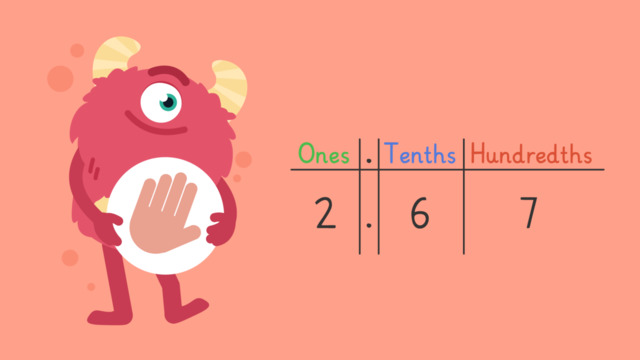# Decimals Greater than 1 as Fractions — Let's Practice!Rating

Ø 3.0 / 4 ratings
The authorsTeam Digital
Decimals Greater than 1 as Fractions — Let's Practice!
CCSS.MATH.CONTENT.4.NF.C.6

## Basics on the topicDecimals Greater than 1 as Fractions — Let's Practice!

Today we are practicing demicals greater than 1 as a fraction with Razzi! This video contains examples to help you further practice and grow confident in this topic.

### TranscriptDecimals Greater than 1 as Fractions — Let's Practice!

Razzi says get these items ready, because today we're going to practice writing decimals greater than one as fractions. It's time to begin! Write two and sixty seven hundredths as a fraction. Pause the video to work on the problem, and press play when you are ready to see the solution! Write each place value as a fraction. Convert the fractions to have the greatest denominator, one hundred. Two ones becomes two hundred hundredths. Six tenths becomes sixty hundredths. Now add the numerators, keeping the denominator the same. Did you also get two hundred sixty-seven hundredths? Let's tackle the next problem! Write six and nine tenths as a fraction. Pause the video to work on the problem, and press play when you are ready to see the solution! Write each place value as a fraction. Convert the fractions to have the greatest denominator, ten. Six ones becomes sixty tenths. Now add the numerators, keeping the denominator the same. Did you also get sixty-nine tenths? Let's tackle the final problem! Write four and one hundredth as a fraction. Pause the video to work on the problem, and press play when you are ready to see the solution! Write each place value as a fraction, ignoring the zero, since it is a placeholder. Convert the fractions to have the greatest denominator, one hundred. Four ones becomes four hundred hundredths. Now add the numerators, keeping the denominator the same. Did you also get four hundred and one hundredths? Razzi had so much fun practicing with you today! See you next time!

## Decimals Greater than 1 as Fractions — Let's Practice! exercise

Would you like to apply the knowledge you’ve learned? You can review and practice it with the tasks for the video Decimals Greater than 1 as Fractions — Let's Practice!.
• ### Match the decimal to a fraction.

Hints

Write each place value as a fraction.

After you write each place value as a fraction, multiply the numerator and denominator so every denominator equals 100. Add the numerators together.

Solution

Write each place value as a fraction.

• For example: 1.67 = $\frac{1}{1}$ + $\frac{6}{10}$ + $\frac{7}{100}$
After you write each place value as a fraction, multiply the numerator and denominator so every denominator equals 100.
• For example: 1.67 = $\frac{100}{100}$ + $\frac{60}{10}$ + $\frac{7}{100}$
When you add all of the numerators, you find the equal fraction.
• For example: 1.67 = $\frac{167}{100}$

• ### True or False?

Hints

Write each place value as a fraction.

Multiply the fractions so they all have a common denominator. Add the numerators together.

Solution

When you write each place value as a fraction and find the common denominator, you can convert the decimal to a fraction.

• ### Write the numerator to solve the equation

Hints

Write each place value as a fraction.

Multiply the fractions so they all have a common denominator, 100. Add the numerators together.

Solution

When you write each place value as a fraction and find the common denominator, 100, you can convert the decimal to a fraction. Add the numerators together and you get 547.

• ### Convert the fraction into a decimal.

Hints

Write each place value as a fraction. When you divide 26 by 10, the 2 is in the ones place and the 6 is in the tens place.

For example, $\frac{26}{10}$ converts to 2.6.

Solution

$\frac{26}{10}$ = 2.6
$\frac{58}{10}$ = 5.8
$\frac{44}{10}$ = 4.4
$\frac{97}{10}$ = 9.7

• ### Match the fraction to the correct decimal.

Hints

Write each place value as a fraction. Make sure to check the tenths and hundredths place.

Multiply the fractions so they all have a common denominator. Add the numerators together.

Solution

When you write each place value as a fraction and find the common denominator, you can convert the decimal to a fraction.

• ### True or False?

Hints

Use the chart to help you. Create fractions for each place value. The next place value after hundredths is thousandths, so the fraction that represents the 9 in the thousandths place is $\frac{9}{1000}$.

The common denominator is 1000. Add the numerators together.

Solution

True. When you convert the decimals into fractions, find the common denominator, and add the numerators together you find that $\frac{2369}{1000}$ = 2.369